# {*no-status title-slide custom-title} // comment- Author: - Supervisors: Rémi Emonet and Marc Sebban - Date:
## Outline - Metric Learning - Examples of Metrics - Mahalanobis distance - Bilinear similarity - Local Metric Learning - Convex Combinations of Local Models - Problem Formulation - Theoretical Guarantees - Applications and Results - Perceptual Color Distance Estimation - Semantic Similarity Estimation - Conclusion

## Metric Learning

### Optimization Problem with similar/dissimilar pairs

\mathrm{arg}\min\:\sum_{m,n} s_{mn}d_A(x_m,x_n) + \lambda \left \|A \right \|^2_F
s.t. \: \sum_{m,n} (1-s_{mn})d_A(x_m,x_n)\geq 1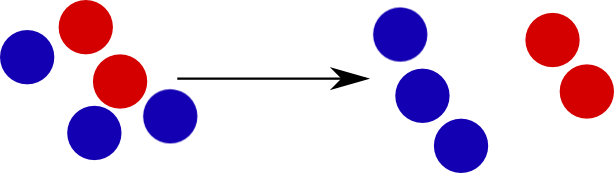where s_{mn} = \begin{cases} 1, & \text{if } x_m, x_n \text{ are similar}\\ 0, & \text{otherwise} \end{cases}

## Metric Functions examples

### Mahalanobis Distance

d_A(x_m,x_n) = \sqrt{(x_m-x_n)^TA(x_m-x_n)}
given
• x_m,x_n two points
• A = S^{-1} (S = covariance matrix)
• A is Positive Semi-Definite (A\succeq0)
• A = L^TL
• d_A(x_m,x_n) = \sqrt{(x_m-x_n)^TL^TL(x_m-x_n)} = \sqrt{(L(x_m-x_n))^T(L(x_m-x_n))}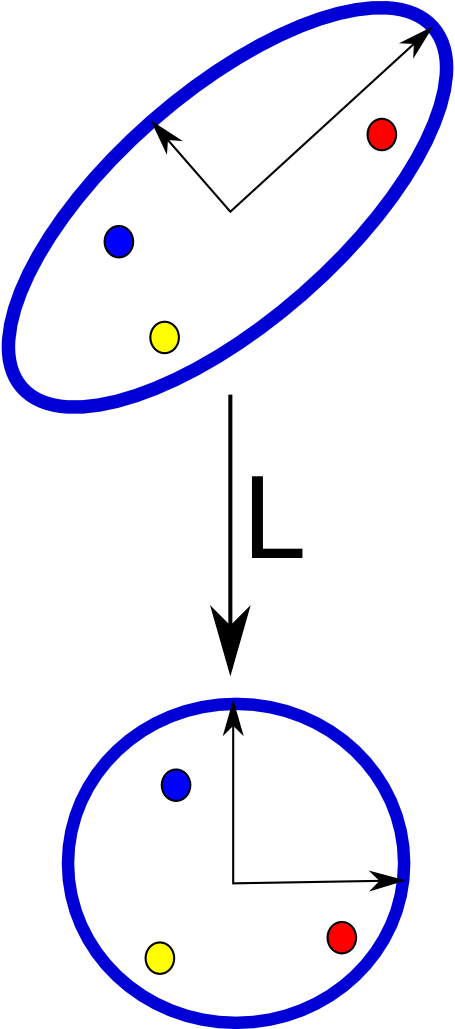## Metric Functions examples

given two points x_m, x_n

### Cosine Similarity

d(x_m,x_n) = \frac{x_m x_n}{||x_m|| ||x_n||} = cos(\theta)

### Bilinear Similarity

d_A(x_m,x_n) = x_m^TAx_n## Global Metric Issues

### Non-linearities and Multi-modalities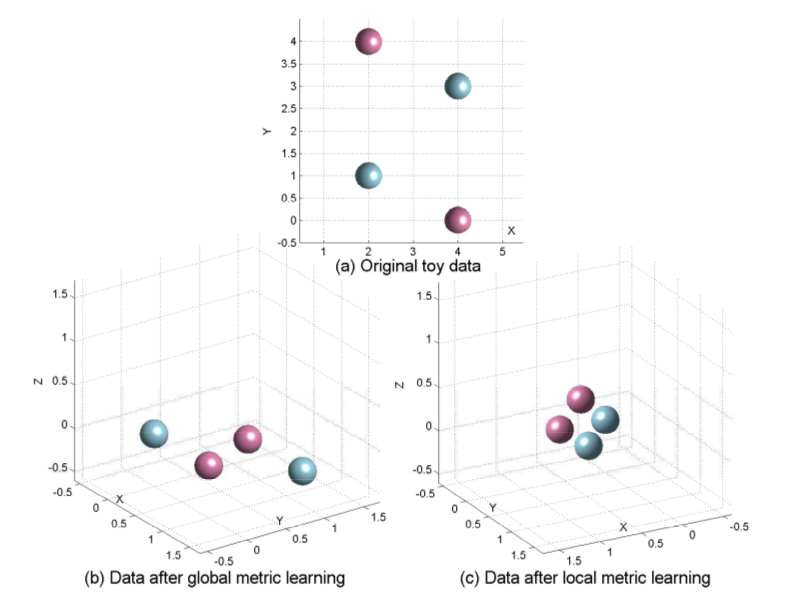Huang, Yinjie, et al. "Reduced-rank local distance metric learning." in Machine Learning and Knowledge Discovery in Databases 2013 ## Local Metric Learning

## Local Metric Learning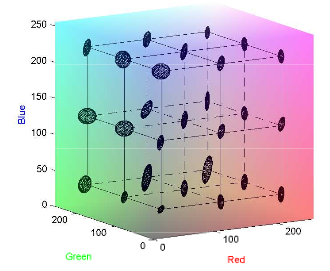1. Kmeans clustering based on Euclidean distance
2. Regression of metrics
• K local metrics
• 1 global metric
+ easy to learn
+ local metrics capture well the underlying geometry of the input space
- high risk of overfitting
- global metric is not enough accurate
Perrot Michaël, Amaury Habrard, Damien Muselet, and Marc Sebban. "Modeling Perceptual Color Differences by Local Metric Learning." In Computer Vision–ECCV 2014
## Outline - Metric Learning - Examples of Metrics - Mahalanobis distance - Bilinear similarity - Local Metric Learning - Convex Combinations of Local Models - Problem Formulation - Theoretical Guarantees - Applications and Results - Perceptual Color Distance Estimation - Semantic Similarity Estimation - Conclusion ## Convex Combinations of Local Metrics

### Convex Combinations

* defined on a clusters' pair $(R_i,R_j)$ * described by a vector $W_{ij} \in \mathbf{R}^K$ representing
the influence of each local metric

$d_{ij}(x_m,x_n) \:=\: \sum_{z}W_{ijz}d_{M_z} (x_m,x_n)$## Convex Combinations of Local Metrics

### Learning Process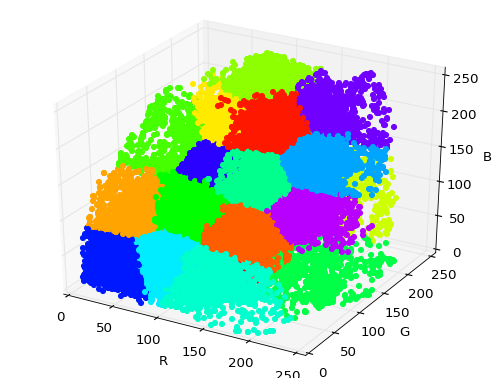KMeans based on Euclidean distance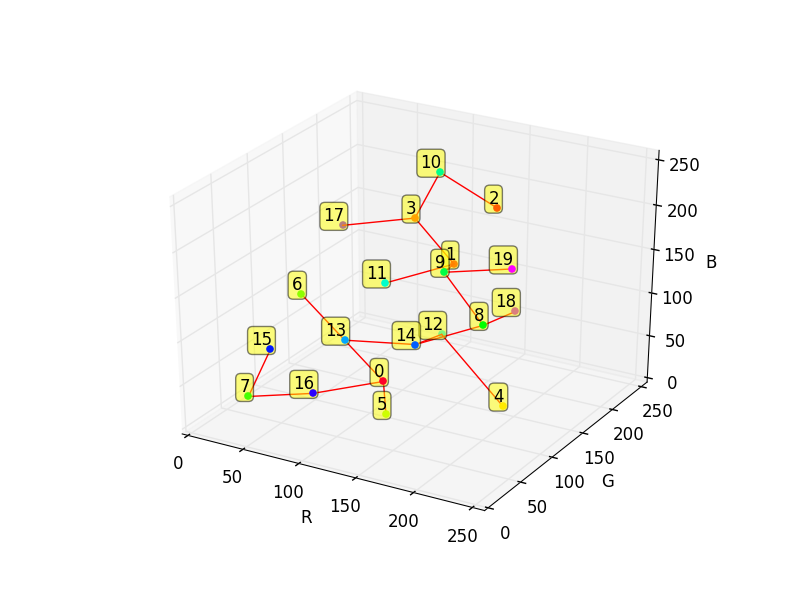between clusters' centers

Find M_z for each cluster

\mathrm{arg}\min_{M_z\succeq0}\:\frac{1}{n_z}\sum_{m,n \in C_z}\left \| d_{M_z}(x_m,x_n) - y(x_m,x_n) \right \| + \left \|M_z \right \|^2_F

## Convex Combinations of Local Metrics

### Learning Convex Combinations

\mathrm{arg}\min_W\: \frac{1}{n}\sum_{i,j}\sum_{(m,n) \in (C_i,C_j)} \left \| d_{ij}(x_m,x_n) - y(x_m,x_n) \right \| \:
+ \: \lambda_{1} D(W) + \: \lambda_{2} S(W)

d_{ij}(x_m,x_n) \:=\: \sum_{z}W_{ijz}d_{M_z} (x_m,x_n)
D(W): topological characteristics of the space's decomposition
S(W): correlation between vectors of weights

## Regularization of the Optimization Problem

### Topological Characteristics of the Space's Decomposition

D(W) = \sum_{i=1}^{Z}\sum_{j=1}^{i-1}\left \| E_{ij}.W_{ij} \right \|_{F}^2E_{65} = \left( \begin{array}{ccc} 4 \\ 6 \\ 4 \\ 6 \\ 2 \\ 2 \\ 2 \\ 8 \\ ... \end{array} \right)

## Regularization of the Optimization Problem

### Correlation between Vectors of Weights

S(W) = \sum_{i=1}^{Z}\sum_{j=1}^{i}\sum_{{i}'=1}^{Z}\sum_{{j}'=1}^{{i}'}K_{ij{i}'{j}'}\left \| W_{ij} - W_{{i}'{j}'}\right \|_{2}^2

## Theoretical Guarantees

### Generalization Bound

difference between

true risk: R^l = \mathbb{E}_{p \sim \mathcal{Z}}\mathcal{l}(W,p)

and

empirical risk: \hat{R}^l = \frac{1}{n} \sum_{p \sim P}\mathcal{l}(W,p)

\left | R^l - \hat{R}^l \right | \leq \phi \left(\frac{\text{VC-dim}}{n}\right)
## Theoretical Guarantees

### Algorithmic Robustness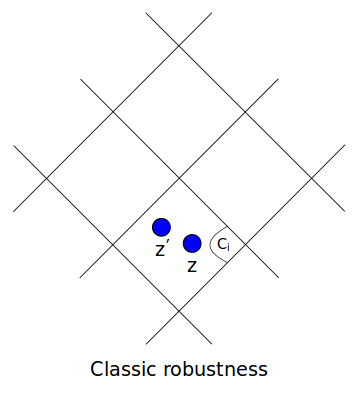Bellet Aurélien and Amaury Habrard. "Robustness and generalization for metric learning." Neurocomputing 151 (2015) ## Theoretical Guarantees with Mahalanobis Distances for any $\delta > 0$ with probability at least   $1-\delta$:
$| R^l - \hat{R}^l | \leq$ $2 \left \|L \right \|_2 \gamma_1 + \gamma_2$ $+$ $B \sqrt{\frac{2H\ln2 + 2\ln{1/\delta}}{n}}$
with - $L$: $M_z = L^TL$ - $\gamma_1$: the radius of the regions defined on the input space - $\gamma_2$: the radius of the regions defined on the output space - $H$: number of regions defined on the input space - $n$: number of instances - $B$: the upper bound of the loss function

## Applications and Results

### Perceptual Color Distanceon RGB space

## Applications and Results

### Perceptual Color Distance Estimation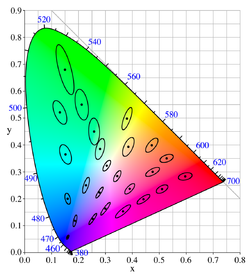### Tolerance

the set of points whose distance to the reference is less than the just-noticeable-difference threshold
can be seen as a set of Mahalanobis metrics

## Applications and Results

### Perceptual Color Distance Estimation

Generalization on unseen colors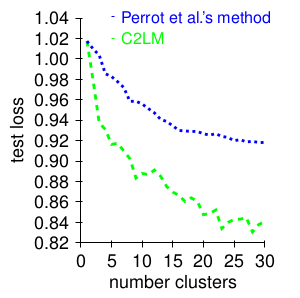Generalization on unseen cameras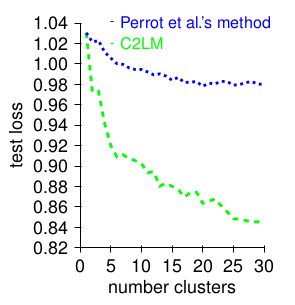## Applications and Results

### Semantic Similarity Estimation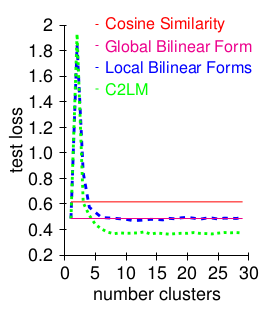Word Embedding computed using Hellinger PCA
Lebret Rémi and Ronan Collobert. "Word emdeddings through hellinger pca." arXiv preprint arXiv:1312.5542 (2013)
## Conclusion - Contributions - Enhancement of local metric approaches by learning Convex Combinations of Local Models - Derivation of Generalization Guarantees through the Algorithmic Robustness framework - Enhancement of Perceptual Color Distance Estimation and Semantic Similarity Estimation Work submitted to CVPR 2016 conference # Thanks for your attention

## Convex Combinations of Local Metrics

### Learning Convex Combinations

S(W) = \sum_{i=1}^{Z}\sum_{j=1}^{i}\sum_{{i}'=1}^{Z}\sum_{{j}'=1}^{{i}'}K_{ij{i}'{j}'}\left \| W_{ij} - W_{{i}'{j}'}\right \|_{2}^2K_{ij,{i}'{j}'} = e^{-min(d_{i{i}'}+d_{j{j}'},d_{i{j}'}+d_{j{i}'})}

K_{56,77} = e^{-2}
K_{56,89} = e^{-9}

/ automatically replaced by the authorautomatically replaced by the title